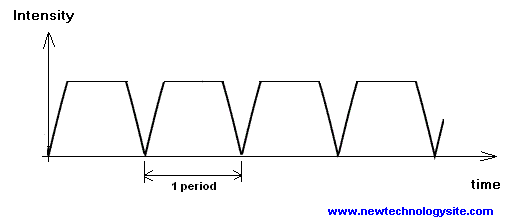# Frequency Unit in SI System

The frequency of periodic signals is explained as well as the units used.

In electronics, signals that repeat in time are called periodic and are characterized by their frequencies. The duration of one cycle is called the period. The frequency of the signal is defined as the number of cycles per unit time. The Hertz is the SI unit defined as the number of cycles (or periods) per second.

If T is the period of the signal in question, then the frequency f is given by

f = 1 / T

For example a signal whose cycle has a duration of 5 seconds, has a frequency f = 1 / 5 = 0.2 Hertz

In electronics however, the duration of one cycle (a period) is very short and is given in microseconds (10-6seconds) and even nanosecond (10-9seconds). A quick calculation gives the corresponding frequencies as 1 / 10-6seconds = 106Hertz which is called 1 Megahertz = 1 MHz and 1 / 10-9seconds = 109Hertz which is called 1 Gigahertz = 1 GHz.

Fig. 1 - Period of Signals

Below is a table of higher frequencies with their names and values.

 Name Symbol Value Kilohertz kHz 103 Hz Megahertz MHz 106 Hz Gigahertz GHz 109 Hz